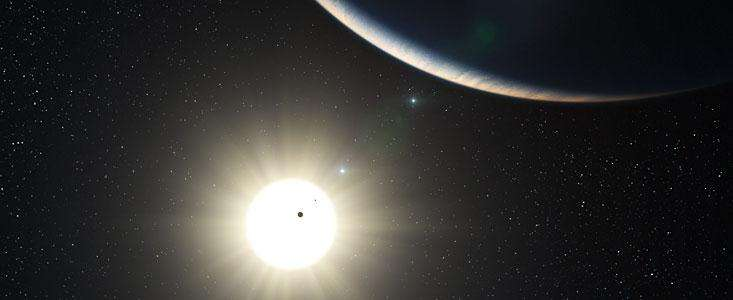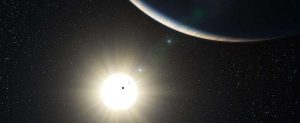# 天文学 TPO2-L4 Bode's Law## TPO2-L4

### Bode’s Law##### Listen to part of a lecture in an astronomy class. You will not need to remember the numbers the professor mentions.

1. What is Bode’s law?
• A. A law of gravitation.
• B. An estimate of the distance between Mars and Jupiter.
• C. A prediction of how many asteroids there are.
• D. A pattern in the spacing of the planets.

1. Why does the professor explain Bode’s Law to the class?
• A. To describe the size of the asteroids.
• B. To explain how the asteroids belt was discovered.
• C. To explain how gravitational forces influence the planets.
• D. To describe the impact of telescopes on astronomy.

1. How does the professor introduce Bode’s Law?
• A. By demonstrating how it is derived mathematically.
• B. By describing the discovery of Uranus.
• C. By drawing attention to the inaccuracy of a certain pattern.
• D. By telling the names of several of the asteroids.

1. According to the professor, what two factors contributed to the discovery of the asteroid Ceres?
• A. Improved telescopes.
• C. The discovery of a new star.
• D. The position of Uranus in a pattern.

1. What does the professor imply about the asteroid belt?
• A. It is farther from the Sun than Uranus.
• B. Bode believed it was made up of small stars.
• C. It is located where people expected to find a planet.
• D. Ceres is the only one of the asteroids that can be seen without a telescope.

1. Why does the professor say this: 🎧

• A. To introduce an alternative application of Bode’s Law.
• B. To give an example of what Bode’s law cannot explain.
• C. To describe the limitations of gravitational theory.
• D. To contrast Bode’s Law with a real scientific law.

D B A AD C D